# Refined Vectors

Online demo

While `rustc` has a keen eye for spotting nasty bugs at compile time, it is not omniscient. We've all groaned in dismay at seeing deployed code crash with messages like

``````panicked at 'index out of bounds: the len is ... but the index is ...'
``````

Next, lets see how flux's refinement and ownership mechanisms let us write a refined vector API whose types track vector sizes and ensure --- at compile time --- that vector accesses cannot fail at runtime.

## Refining Vectors to Track their Size

To begin with, we will defined a refined vector type which is simply a wrapper around the standard `Vec` type

``````#![allow(unused)]
fn main() {
#[flux::refined_by(len: int)]
pub struct RVec<T> {
inner: Vec<T>,
}
}``````

The `#[flux::refined_by(len: int)]` attribute tells flux that the type `RVec<T>` struct is indexed by a `len` refinement which tracks the size of the underlying vector, just like the indices for `i32` and `bool` tracked the actual value of the underlying integer or boolean). The idea is that the type

• `RVec<i32>` represents a vector of `i32` size 10, and
• `RVec<bool>{v:0 < v}` represents a non-empty vector of `bool`, and
• `RVec<RVec<f32>[n]>[m]` represents a vector of vectors of `f32` of size `m` and each of whose elements is a vector of size `n`.

### Creating Vectors

Now that we can talk about the size of a vector, lets build up an API for creating and manipulating vectors. I suppose one must start with nothing: an empty vector.

``````#![allow(unused)]
fn main() {
impl<T> RVec<T> {
#[flux::trusted]
#[flux::sig(fn() -> RVec<T>)]
pub fn new() -> Self {
Self { inner: Vec::new() }
}
}
}``````

The above implements `RVec::new` as a wrapper around `Vec::new`. The `#[flux::trusted]` attribute tells Flux there is nothing to "check" here, as we are defining the API itself and trusting that the implementation (using `vec` is correct). However, the signature says that callers of the `RVec::new` get back a vector indexed with `0` i.e. an empty vector.

### Pushing Values

An empty vector is a rather desolate thing.

To be of any use, we need to be able to `push` values into the container, like so

``````#![allow(unused)]
fn main() {
#[flux::trusted]
#[flux::sig(fn(self: &strg RVec<T>[@n], T)
ensures self: RVec<T>[n+1])]
pub fn push(&mut self, item: T) {
self.inner.push(item);
}
}``````

The refined type for `push` says that it takes a strong reference (`self`) --- where `strg` means the refined type may be changed by the function --- to an `RVec<T>` of size `n` and a value `T` and upon exit, the size of `self` is increased by `1`.

### Popping Values

Not much point stuffing things into a vector if we can't get them out again.

For that, we might implement a `pop` method that returns the last element of the vector. Aha, but what if the vector is empty? You could return an `Option<T>` or since we're tracking sizes, we could require that `pop` only be called with non-empty vectors.

``````#![allow(unused)]
fn main() {
#[flux::trusted]
#[flux::sig(fn(self: &strg {RVec<T>[@n] | 0 < n}) -> T
ensures self: RVec<T>[n-1])]
pub fn pop(&mut self) -> T {
self.inner.pop().unwrap()
}
}``````

### Using the API

Now already `flux` can start checking some code, for example if you `push` two elements, then you can `pop` twice, but flux will reject the third `pop` at compile-time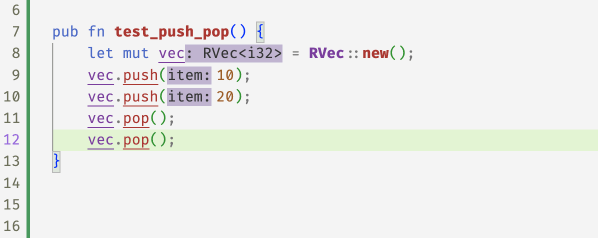In fact, the error message from `flux` will point to exact condition that does not hold

``````#![allow(unused)]
fn main() {
error[FLUX]: precondition might not hold
--> src/vectors.rs:24:5
|
24 |     v.pop();
|     ^^^^^^^ call site
|
= note: a precondition cannot be proved at this call site
note: this is the condition that cannot be proved
--> src/rvec.rs:78:47
|
78 |     #[flux::sig(fn(self: &strg {RVec<T>[@n] | 0 < n}) -> T
|                                               ^^^^^
}``````

### Querying the Size

Perhaps we should peek at the size of the vector to make sure its not empty before we `pop` it. We can do that with a `len` method whose type says that the returned `usize` is, in fact, the size of the input vector

``````#![allow(unused)]
fn main() {
#[flux::trusted]
#[flux::sig(fn(&RVec<T>[@n]) -> usize[n])]
pub fn len(&self) -> usize {
self.inner.len()
}
}``````

Now, flux "knows" that after two `push`es, the size of the vector is `2` and after the two `pop`s, the size is `0` again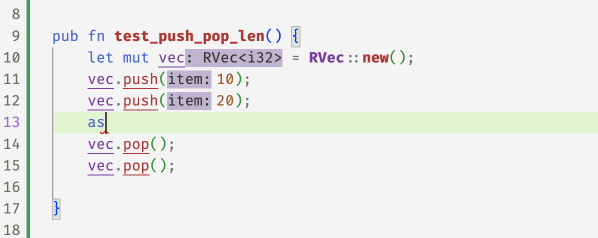### Random Access

Of course, vectors are not just stacks, they also allow random access to their elements which is where those pesky panics occur, and where the refined vector API gets rather useful. Since we're tracking sizes, we can require that the method to `get` an element only be called with a valid index that is between `0` and the vector's size

``````#![allow(unused)]
fn main() {
#[flux::sig(fn(&RVec<T>[@n], i: usize{i < n}) -> &T)]
pub fn get(&self, i: usize) -> &T {
&self.inner[i]
}

#[flux::sig(fn(&mut RVec<T>[@n], i: usize{i < n}) -> &mut T)]
pub fn get_mut(&mut self, i: usize) -> &mut T {
&mut self.inner[i]
}
}``````

With these refined `get` methods, flux can now spot the ``off-by-one'' error in the following code and accepts the fix 1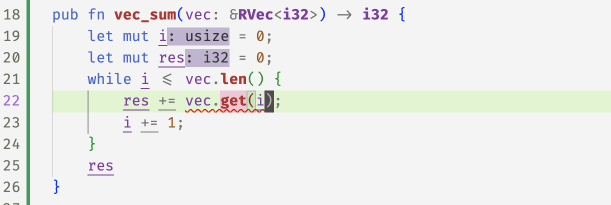Its a bit gross to use `get` and `get_mut` directly, so instead we implement the `Index` and `IndexMut` traits for `RVec` which allows us to use the `[]` operator to access elements

``````#![allow(unused)]
fn main() {
impl<T> std::ops::Index<usize> for RVec<T> {
type Output = T;
#[flux::sig(fn(&RVec<T>[@n], i:usize{i < n}) -> &T)]
fn index(&self, index: usize) -> &T {
self.get(index)
}
}

impl<T> std::ops::IndexMut<usize> for RVec<T> {
#[flux::sig(fn(&mut RVec<T>[@n], i:usize{i < n}) -> &mut T)]
fn index_mut(&mut self, index: usize) -> &mut T {
self.get_mut(index)
}
}
}``````

And now the above `vec_sum` example looks a little nicer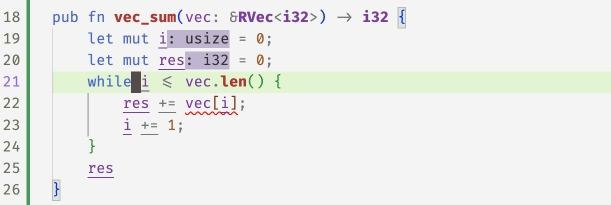## Memoization

Lets put the whole API to work in this "memoized" version of the fibonacci function which uses a vector to store the results of previous calls

``````#![allow(unused)]
fn main() {
pub fn fib(n: usize) -> i32 {
let mut r = RVec::new();
let mut i = 0;
while i < n {
if i == 0 {
r.push(0);
} else if i == 1 {
r.push(1);
} else {
let a = r[i - 1];
let b = r[i - 2];
r.push(a + b);
}
i += 1;
}
r.pop()
}
}``````

Oops, flux is not happy with the call to `pop` at the end of the function which returns the last value as the result.

``````#![allow(unused)]
fn main() {
error[FLUX]: precondition might not hold
--> src/vectors.rs:40:5
|
40 |     r.pop()
|     ^^^^^^^
}``````

Flux complains that the vector may be empty and so the `pop` call may fail ... but why? Can you spot the problem?

Indeed, we missed a "corner" case -- when `n` is `0` we skip the loop and so the vector is empty! Once we add a test for that, flux is happy.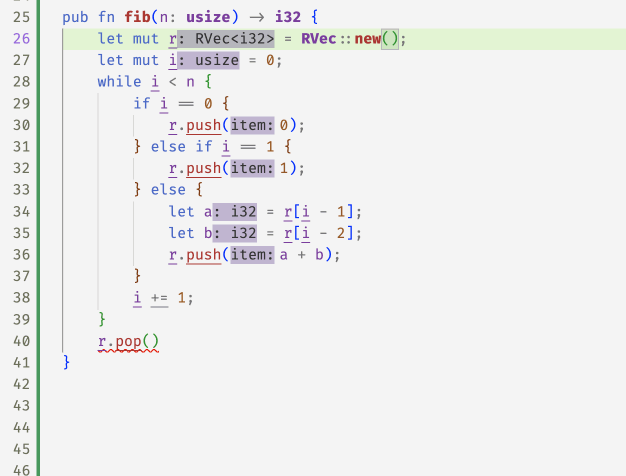As a last example, lets look at a simplified version of the `binary_search` method from `std::vec`, into which I've snuck a tiny little bug

``````#![allow(unused)]
fn main() {
pub fn binary_search(vec: &RVec<i32>, x: i32) -> Result<usize, usize> {
let mut size = vec.len();
let mut left = 0;
let mut right = size;
while left <= right {
let mid = left + size / 2;
let val = vec[mid];
if val < x {
left = mid + 1;
} else if x < val {
right = mid;
} else {
return Ok(mid);
}
size = right - left;
}
Err(left)
}
}``````

Flux complains in two places

``````#![allow(unused)]
fn main() {
error[FLUX]: precondition might not hold
--> src/vectors.rs:152:19
|
152 |         let val = vec[mid];
|                   ^^^^^^^^ call site
|
= note: a precondition cannot be proved at this call site
note: this is the condition that cannot be proved
--> src/rvec.rs:189:44
|
189 |     #[flux::sig(fn(&RVec<T>[@n], usize{v : v < n}) -> &T)]
|                                            ^^^^^

error[FLUX]: arithmetic operation may overflow
--> src/vectors.rs:160:9
|
160 |         size = right - left;
|         ^^^^^^^^^^^^^^^^^^^
}``````
• The vector access may be unsafe as `mid` could be out of bounds!

• The `size` variable may underflow as `left` may exceed `right`!

Can you the spot off-by-one and figure out a fix?

## Summary

So, we saw how Flux's index and constraint mechanisms combine with Rust's ownership to let us write a refined vector API that ensures the safety of all accesses at compile time.

Next time, we'll see how these mechanisms are compositional in that we can use standard type machinery to build up compound structures and APIs from simple ones.

1

Why not use an iterator? We'll get there in due course!